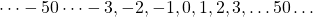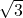# Number Classification

Number classifications allow us to describe numbers efficiently.

# Counting Chairs and Measuring Plants

The number of chairs in a classroom is usually between 0 and 30. Letrepresent the number of chairs in a classroom, then we can use this notation:Let’s be more precise. We mean between 0 and 30, whole numbers only. ThereforeThe letter-like-symbolis used for natural numbers – that is – positive whole numbers.

On the other hand, if I am measuring an amaryllis plant that will grow to 60 cm, I can say that during its growth period it is is between zero and 60 cm.In this case, it will grow through all possible values. Letrepresent the height of an amaryllis plant in cm, and we can sayThe letter-like-symbolis used for real numbers – that is – all numbers represented on a continuous number line from negative infinity to positive infinity.

# The Default

If no number set is mentioned when defining the domain of a function it is assumed that the set intended is the real number set.

# The four major number sets

In this course, you are expected to be familiar with Natural Numbers, Integers, Rational Numbers and Real Numbers. Each of these sets of numbers has a symbol – a letter like symbol – that we use as shorthand.

Natural numbers:Integers:Rational Numbers:Real Numbers:Natural numbers: whole numbers greater than zero. For exampleetc. Sometimesis included in this set.

Integers: positive and negative whole numbers and zero. For exampleRational numbers: all numbers that can be written as a fraction with whole numbers for numerator and denominator. That is, allwhere bothandare integers with the exception thatcannot be zero.

Real numbers: Rational and Irrational numbers, where irrational numbers are values that exist on the number line but cannot be written as a fraction.

# Venn Diagram

A venn diagram of these sets shows that the sets are layered. (Image source)• all natural numbers are integers,
• All integers are rational,
• All rational numbers are real,

However

• some real numbers are not rational, (for example)
• some rational numbers are not integers, (for example,)
• some integers are not natural numbers. (for example,).

# Irrational Numbers

Irrational numbers are numbers that can’t be written as a fraction.andare two examples. In fact, any square root of a non-square number is irrational.

It was once believed that all numbers were rational. The discovery that it is impossible to write some known quantities as a ratio of two integers was upsetting to the mathematical community at that time. This video describes the upset that irrational numbers caused when they became known.